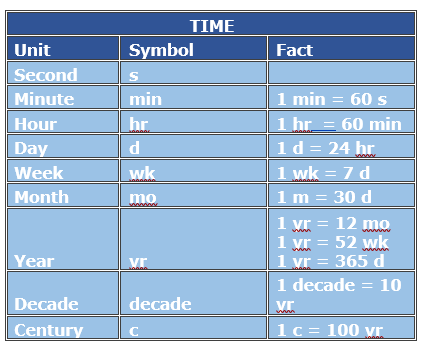# Time Unit Conversion with Whole Number Values

#### Complete Python Prime Pack

9 Courses     2 eBooks

#### Artificial Intelligence & Machine Learning Prime Pack

6 Courses     1 eBooks

#### Java Prime Pack

9 Courses     2 eBooks

The basic metric unit for time is a second. Other metric units for time are minutes, hours, days and so on. These units of time are same as the US customary units of time.

In this lesson, we solve problems on conversion among various metric units for time involving whole number values only.Fill in the blank to make the conversion true.

90 days = __ months

### Solution

Step 1:

We know that

30 days = 1 month

Step 2:

90 days = 90 days × 1 month/30 days = 3 months

So, 90 days = 3 months

Fill in the blank to make the conversion true.

4800 seconds = __ minutes

### Solution

Step 1:

We know that

60 seconds = 1 minute

Step 2:

4800 seconds = 4800 seconds × 1 minutes/60 seconds = 80 minutes

So, 4800 seconds = 80 minutes

Fill in the blank to make the conversion true.

4 years = __days

### Solution

Step 1:

We know that

1 year = 365 days

Step 2:

4 years = 4 years × 365 days/1 year = 1460 days

So, 4 years = 1460 days